# 2295: Garbage Math

 Garbage MathTitle text: 'Garbage In, Garbage Out' should not be taken to imply any sort of conservation law limiting the amount of garbage produced.

## ExplanationThis explanation may be incomplete or incorrect: Created by a ZILOG Z80. Please mention here why this explanation isn't complete. Do NOT delete this tag too soon.If you can address this issue, please edit the page! Thanks.

This comic explains the "garbage in, garbage out" concept using arithmetical expressions. Just like the comic says, if you get garbage in any part of your workflow, you get garbage as a result.

Some of these rules correspond to the rules of floating point arithmetic, while others may be inspired by the rules of propagation of uncertainty where a "garbage" number would correspond to an estimate with a high degree of uncertainty, and the uncertainty of the result of arithmetic operations will tend to be dominated by the term with the highest uncertainty. The rule about N pieces of independent garbage reflects the central limit theorem and how it predicts that the uncertainty (or standard error) of an estimate will be reduced when independent estimates are averaged. The comic oddly omits raising garbage to the 0th power, which transforms even NaN, the platonic ideal of garbage, to exactly 1.

This comic is not related to the 2020 pandemic of the coronavirus SARS-CoV-2, which causes COVID-19, breaking the streak of comics preceding this on topics relating to COVID-19, after (rather appropriately) 19 comics (not counting the April Fools' comic).

This comic is about the propagation of errors in numerical analysis and statistics, but described in much more colloquial terms. Numbers with low precision are termed "garbage" and numbers with high precision are labeled "precise".

Formula Statistical Expression Explanation
Precise number + Precise number = Slightly less precise number$\mathop\sigma(X+Y)=\sqrt{(\mathop\sigma(X))^2+(\mathop\sigma(Y))^2}$ If we know absolute error bars, then adding two precise numbers will at worst add the sizes of the two error bars. For example, if our precise numbers are 1 (±10-6) and 1 (±10-6), then our sum is 2 (±2·10-6). It is possible to lose a lot of relative precision, if the resultant sum is close to zero as a result of adding a number and then close to its inverse. This phenomenon is known as catastrophic cancellation. Therefore, it is likely that all numbers referred here are positive numbers, which does not exhibit this phenomenon.
Precise number × Precise number = Slightly less precise number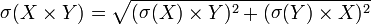$\mathop\sigma(X\times Y)=\sqrt{(\mathop\sigma(X)\times Y)^2+(\mathop\sigma(Y)\times X)^2}$ Here, instead of absolute error, relative error will be added. For example, if our precise numbers are 1 (±10-6) and 1 (±10-6), then our product is 1 (±2·10-6).
Precise number + Garbage = Garbage$\mathop\sigma(X+Y)=\sqrt{(\mathop\sigma(X))^2+(\mathop\sigma(Y))^2}$ If one of the numbers has a high absolute error, and the numbers being added are of comparable size, then this error will be propagated to the sum.
Precise number × Garbage = Garbage$\mathop\sigma(X\times Y)=\sqrt{(\mathop\sigma(X)\times Y)^2+(\mathop\sigma(Y)\times X)^2}$ Likewise, if one of the numbers has a high relative error, then this error will be propagated to the product. Here, this is independent of the sizes of the numbers.$\sqrt{\text{Garbage}} = \text{Less bad garbage}$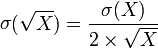$\mathop\sigma(\sqrt X)=\frac{\mathop\sigma(X)}{2\times\sqrt X}$ When the square root of a number is computed, its relative error will be halved. Depending on the application, this might not be all that much better, but it's at least less bad.
Garbage2 = Worse garbage$\mathop\sigma(X^2)=2\times X\times\mathop\sigma(X)$ Likewise, when a number is squared, its relative error will be doubled. This is a corollary to multiplication adding relative errors.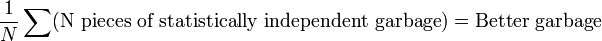$\frac{1}{N}\sum(\text{N pieces of statistically independent garbage}) = \text{Better garbage}$ By aggregating many pieces of statistically independent observations (for instance, surveying many individuals), it is possible to reduce relative error. This is the basis of statistical sampling.
Precise numberGarbage = Much worse garbage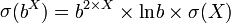$\mathop\sigma(b^X)=b^{2\times X}\times\mathop{\mathrm{ln}}b\times\sigma(X)$ The exponent is very sensitive to changes, which may also magnify the effect based on the magnitude of the precise number.
Garbage – Garbage = Much worse garbage$\mathop\sigma(X-Y)=\sqrt{(\mathop\sigma(X))^2+(\mathop\sigma(Y))^2}$ This line involves catastrophic cancellation. If both pieces of garbage are about the same (e.g. if their error bars overlap), then it is possible that the answer is positive, zero, or negative.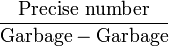$\frac{\text{Precise number}}{\text{Garbage}-\text{Garbage}}$ = Much worse garbage, possible division by zero$\mathop\sigma(\frac{X}{Y})=\sqrt{\mid{(\mathop\sigma(X)\times Y)^2-(\mathop\sigma(Y)\times X)^2}\mid}$ Indeed, as with above, if error bars overlap then we might end up dividing by zero.
Garbage × 0 = Precise number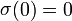$\mathop\sigma(0)=0$ Multiplying anything by 0 results in 0, an extremely precise number in the sense that it has no error whatsoever since we supply the 0 ourselves. This is equivalent to discarding garbage data from a statistical analysis.

The title text refers to the computer science maxim of "garbage in, garbage out," which states that when it comes to computer code, supplying incorrect initial data will produce incorrect results, even if the code itself accurately does what it is supposed to do. As we can see above, however, when plugging data into mathematical formulas, this can possibly magnify the error of our input data, though there are ways to reduce this error (such as aggregating data). Therefore, the quantity of garbage is not necessarily conserved.

## TranscriptThis transcript is incomplete. Please help editing it! Thanks.

[A series of mathematical equations are written from top to bottom]

Precise number + Precise number = Slightly less precise number

Precise number × Precise number = Slightly less precise number

Precise number + Garbage = Garbage

Precise number × Garbage = Garbage

1/N Σ (N pieces of statistically independent garbage) = Better garbage

(Precise number)Garbage = Much worse garbage

Garbage – Garbage = Much worse garbage

Precise number / ( Garbage – Garbage ) = Much worse garbage, possible division by zero

Garbage × 0 = Precise numberadd a comment! ⋅add a topic (use sparingly)! ⋅refresh comments!

# Discussion

## Inclusion in Series

This is not a Covid19 comic. One could think that this is a comment on the difficulties of modeling the corona virus outbreak, but since discussions of exponential functions are only a small part in the comic I believe it is just a general comment on floating point arithmetic mixed in with statistical considerations. --108.162.229.242 17:28, 17 April 2020 (UTC)

I disagree that this is not a COVID-19 comic. I also believe the one about visualizing large numbers was COVID-19 related. On the other hand, I like the idea that Randall might produce exactly 19 comics related to SARS CoViD 2019, so I'm prepared to concede the point for the sake of arbitrary numerological appeal.
ProphetZarquon (talk) 18:42, 17 April 2020 (UTC)
I think Exa-Exabyte was a real stretch (the virus doesn't even have DNA), but there is a tenuous link so whatever. The idea that this comic is related, on the other hand, stretches past the breaking point. There's hardly anything that can't be linked to global events if we try hard enough, but that doesn't mean there's an actual link. Sometimes a comic about garbage math is just a comic about garbage math. 172.69.71.58 19:33, 17 April 2020 (UTC)
I think this one's much more likely to be a coronavirus comic than Exa-Exabyte was. There's an awful lot of COVID data, much of it either very imprecise or outright garbage; and the comic directly before this one (2294) involved bad modeling of said COVID data, so clearly COVID data (and its limitations) is something Randall's currently thinking of and drawing comics about. Pelosujamo (talk) 20:25, 17 April 2020 (UTC)
Exa-Exabyte was centered around biology, which gives reason to believe it was covid19 related. This one seems much more uncertain. Any conclusion that it is related is based on garbage. Jokes aside, It seems like much more of a stretch to me. Randall thinking in those terms is a reasonable argument, but personally I am going to assume this is the chain breaker unless a direct reference is made in the next couple comics since ending at 19 is would be appropriate. 172.69.70.209
While this comic has no direct reference to Covid-19 it does appear that the math might be related. At this point we can't know if the series has ended. As such I've edited the paragraph in the explanation to identify the known ambiguities. And now I realize I've made an explanatory paragraph about "knowledge error bars" in the explanation of a comic about numerical error bars.Iggynelix (talk) 14:42, 18 April 2020 (UTC)
No. The reason it appears the math might be related is because the math relates to everything, everywhere. That's not enough of a connection. During this pandemic, there will be a lot of comics related to the coronavirus, many of them in a row, but that doesn't mean that every comic that could be tangentially related if you squint just right should qualify as a COVID-19 comic (I still think Exa-Exabyte doesn't). There needs to be a real link, because just about anything could be twisted into a relation if you try hard enough. As a test, I hit Special:Random and got 346: Diet Coke+Mentos. Wouldn't you know, that's a coronavirus comic! The father, you see, actually had COVID-19 and died, but Diet Coke and Mentos has brought him back! No. The line should be drawn here. The streak has ended. 172.69.68.197 17:02, 18 April 2020 (UTC)
I agree this is not a serious contender for inclusion as a COVID comic. Although I'm pretty sure Randall has input to COVID19 models as garbage on his mind. But there is nothing in this comic that suggest this math be used on a pandemic. The exa byte is a different story as it is about how much of biology we cannot know or control in the midst of a lot of comics about some new biology we do not control. I do not expect that this will end the covid19 series, but I will consent that even if the next comic is a clear corona comic, it will no longer be an unbroken streak. Anyway the real streak ended at the end of March with the late April Fool's comic. I also do not at all think that the coke mentos could be seen as a COVID19 comic, that is just bulls**t trying to prove a point that I believe you fail completely. I also tried random comic (I like the idea) and found 1208: Footnote Labyrinths. It is a scientific paper (with nested footnotes) and given science, we could say it was about science about Corona. Naah. But for the same reason this comic should not be considered corona. --Kynde (talk) 20:53, 18 April 2020 (UTC)
I am pretty sure this IS related. Right now, everybody and his grandmother is staring at the Johns Hopkins Coronavirus numbers for different countries. Entire newspaper articles are written about these numbers and about why one country is apparently faring better than the other and what this means. The numbers are made into fancy graphics. People use these numbers to calculate fatality rates and cure rates. Politicians might even use these numbers to make decisions.
And all this even though everybody KNOWS that the numbers cannot really be compared from one country to the other, because testing prerequisites vary, testing availability varies, testing procedures vary, criteria used to include a death as a coronavirus death vary. The sources of the numbers are very different and might not always be reliable. [Apparently, they include local language newspapers, website and even social media accounts. How many people DOES the Johns Hopkins University have to track all these sources reliably, worldwide, in local languages?] And not to forget some countries probably are downright lying.
And still, people are comparing. I've read articles where the author admits the numbers are probably garbage in one sentence and then STILL goes on to calculate fatality rates from them in the next sentence. So, most PROBABLY related.
I challenge you to find a comic in the archive that can't be twisted to say it's related to COVID-19. At this point people are finding connections in the same way that people analyze "the curtain is blue". 108.162.245.26 22:06, 19 April 2020 (UTC)

--141.101.69.153 21:53, 19 April 2020 (UTC)

Don't you mean the dress is blue?
I think this is more SARS-CoV-2 related than exa-exa (or Conway), but the desire is for there to have been 19 in a row, so there were 19 in a row. No doubt the next strip will be seen as the first in a second run of 19 162.158.34.222 23:33, 23 April 2020 (UTC)

This comic very much reminds me of this article: [https://www.realclearmarkets.com/articles/2020/04/17/its_decidedly_not_the_math_its_always_people_489344.html It's Decidedly Not the Math. It's Always People] So much so that my first thought was that the comic was inspired by it, though of course I can't prove it.BrianZ (talk) 00:52, 20 April 2020 (UTC)

## Math and Error bars

Well this is surprising came here thinking I understood it just to see what the discussion looked like. Ended up learning something new. I was able to understand intuitively the comic. But this is my first exposure to actually doing math on the error bars. I think I was supposed to do that in college but I don't remember anyone ever explaining how it should work. --162.158.63.208 18:14, 17 April 2020 (UTC)

In recent days, there have been a number of math "quizzes" in this same type of format, albeit generally with only addition and maybe multiplication, appearing on Facebook. Should the explanation include a reference to this as a possible contributing reason for Randall's comic? One could also argue that those quizzes have been appearing on Facebook as a way to spend/waste time during the coronavirus pandemic lock-down, making he comic at least tangentially related to Covid19 LIES.

Unsigned vandalism? /\ change history @user Please feel free to move your discussion to an appropriate forum and remove both the edit and this comment at such time. Iggynelix (talk)

What's the difference between relative error and absolute error? I don't understand these terms. Maybe add?

Absolute error is the amount of uncertainty in a value measured as a given number. e.g. 5.7 ± 1.2 means that actual value lies somewhere between 5.7 - 1.2 and 5.7 + 1.2 = 4.5 to 6.9. If you change the 5.7 to another value, you still get the same absolute difference of maximum and minimum values. Relative error depends on the value you are comparing to. e.g. 5.7 ± 10% would be between 5.7 - 0.57 and 5.7 + 0.57 = 5.13 to 6.27. The absolute difference of maximum and minimum would change if the main number changes. e.g. 11.3 ± 10% would be between 10.17 and 12.43, which has a greater absolute difference of maximum and minimum than the previous example. Nutster (talk) 01:54, 18 April 2020 (UTC)

Are all of these equations consistent with garbage = infinity?

Unfortunately, as written, these equations would not make sense by defining Garbage as an infinity. Infinity is not a number you can count to or measure in between integers. Infinity is the idea of unending-ness. Trying to use infinity as if it a finite number yields all sorts of invalid results. In this case Garbage is defined as an arbitrary finite number with a large amount of uncertainty in its value. Nutster (talk) 01:40, 18 April 2020 (UTC)
That's a pretty good definition of 'garbage' in any case, plus or minus 10%. ( See also valuable garbage) Iggynelix (talk) 14:19, 18 April 2020 (UTC)

Would the summation divided by n just give you the arithmatic mean of the data set? Nutster (talk) 01:55, 18 April 2020 (UTC)

Pretty much, but the point is probably more that (without consistent bias across the set, just 'random' errors for each item) it suppresses the degree of garbagicity as outliers are increasingly nullified by the greater number of more competently accurate values and (if it's a symmetric error) opposing outliers. 162.158.34.222 09:29, 18 April 2020 (UTC)

The statement that NaN^0 isn't fully justified and I'm not clear it belongs. Djbrasier (talk) 18:46, 18 April 2020 (UTC)

I agree... It also isn't evident to me that this comic has anything to do with floating-point math, which is the only thing that could (even slimly) justify its inclusion. This is about statistics, not programming. --108.162.215.12 05:25, 19 April 2020 (UTC)

I'm concerned that, with "Precise Number" there's the usual confusion between Accuracy and Precision (edit: and of course Resolution, too!). A precise number can still be utter garbage, as 84.7489327(646475)% of all mathematicians could tell you. 162.158.111.241 13:59, 19 April 2020 (UTC)

The table of formulae for the propagation of variance σ addresses that aspect. You can't know the accuracy of a result without knowing the precision of its calculation, and while reducing precision always reduces accuracy, it's not the other way around. But precision is inherent in the representation and operations, while accuracy is secondary when you aren't discussing the initial measurements of the inputs, so I think the terminology is correct.
By the way, shout out to 172.68.51.124 for filling out all but one of those table entries. I wonder where they looked them up. I'm guessing a CRC Handbook left over from High School chemistry or some such? Anyway, good job! This really looks classy now that it's been cleaned up a bit. 162.158.255.64 06:45, 20 April 2020 (UTC)

Could someone please double check that the given uncertainty formula for "Precise number / ( Garbage – Garbage )" at the second to the bottom is correct? I'm not sure it properly accommodates the uncertainty of the numerator. 162.158.255.64 07:48, 20 April 2020 (UTC)

Are the changes from "=" to "≈" correct? Either way, isn't the proper symbol for the relation "≅" ("approximately equal to") instead of "≈" ("almost equal to")? As is illustrated by catastrophic cancellation, an approximation may not be "almost" correct. But my question is, aren't those relations to the resulting standard deviation exact instead of approximate? 172.69.22.152 04:16, 22 April 2020 (UTC)

The formulas are the first approximation for small sigma. They are exact for a linear combination of the random variables in the term. With rising sigma, higher order terms can get relevant. Sebastian --172.69.54.141 07:46, 23 April 2020 (UTC)

Are the results truly correct? Wouldn't the final sum and product standard deviations be √2 10^6 ?

This comic makes me think about the "Garbage In, Garbage Out" rule of programming as well. Probably unrelated, but it just came to mind. Sarah the Pie(yes, the food) (talk) 11:14, 9 May 2021 (UTC)